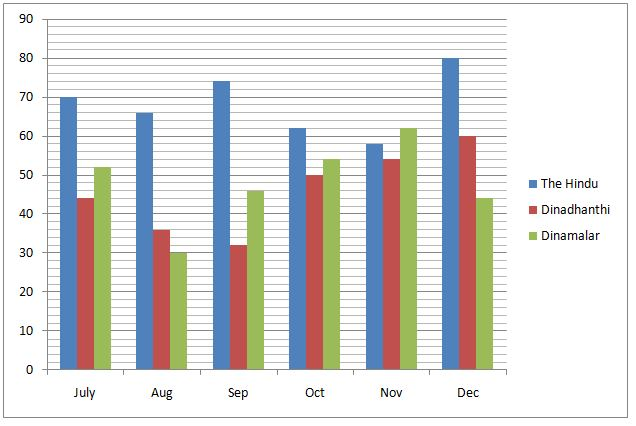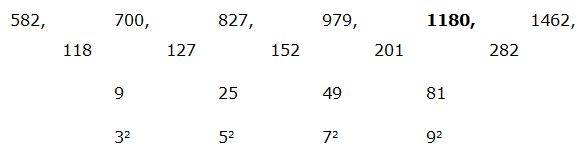# CWC/FCI Prelims 2019 – Quantitative Aptitude Questions (Day-08)

Dear Readers, Exam Race for the Year 2019 has already started, To enrich your preparation here we are providing new series of Practice Questions on Quantitative Aptitude – Section for CWC/FCI Exam. Aspirants, practice these questions on a regular basis to improve your score in aptitude section. Start your effective preparation from the right beginning to get success in upcoming CWC/FCI Exam.

[WpProQuiz 5469]

Directions (Q. 1 – 5): Find the wrong term in the following number series?

1) 273, 277, 281, 293, 313, 343

a) 277

b) 313

c) 343

d) 281

e) 293

2) 8748, 2916, 978, 324, 108, 36

a) 324

b) 2916

c) 108

d) 978

e) 36

3) 72, 37, 38, 58, 117, 293, 881.5

a) 38

b) 293

c) 117

d) 881.5

e) 72

4) 582, 700, 827, 979, 1178, 1462

a) 827

b) 979

c) 1178

d) 1462

e) 1765

5) 340, 2040, 408, 1632, 542, 1088

a) 542

b) 408

c) 1632

d) 1088

e) 340

Directions (Q. 6 – 10): Study the following information carefully and answer the given questions:

The following bar graph shows the total number of 3 different types of newspapers (In lakhs) printed on 6 different months in the year 2018 in a certain state. (Only Two languages viz. English and Tamil newspaper)6) Find the ratio between the total number of all the given newspapers printed in the month of Aug to that of Nov?

a) 11: 17

b) 35: 44

c) 22: 29

d) 52: 55

e) None of these

7) If the percentage of total number of English newspaper printed in the month of Aug and Oct by Dinamalar is 30 % and 25 % respectively, then find the difference between the total number of Tamil newspaper printed in the month of Aug to that of Oct by Dinamalar?

a) 19.5 lakhs

b) 22 lakhs

c) 18 lakhs

d) 23.5 lakhs

e) None of these

8) Find the average number of Dinadhanthi newspapers printed on all the given months together?

a) 52 lakhs

b) 48 lakhs

c) 50 lakhs

d) 46 lakhs

e) None of these

9) Total number of all the given newspapers printed in the month of July and Sep together is approximately what percentage of total number of Dinamalar newspaper printed on all the given months together?

a) 85 %

b) 110 %

c) 125 %

d) 70 %

e) 140 %

10) Find the difference between the total number of the Hindu newspaper to that of Dinamalar newspaper printed on all the given months together?

a) 145 lakhs

b) 136 lakhs

c) 122 lakhs

d) 108 lakhs

e) None of these

Directions (Q. 1-5):

The correct series is,

273, 275, 281, 293, 313, 343

The pattern is, + (12 + 1), + (22 + 2), + (32 + 3), + (42 + 4),….

The wrong term is, 277

The correct series is,

8748, 2916, 972, 324, 108, 36

The pattern is, ÷ 3

The wrong term is, 978

The correct series is,

72, 37, 38, 58, 117, 293.5, 881.5

The pattern is, *0.5 + 1, *1 + 1, *1.5 + 1, *2 + 1, *2.5 + 1, *3 + 1,….

The wrong term is, 293

The correct series is,The difference of difference is, 32, 52, 72, 92,….

The wrong term is, 1178

The correct series is,

340, 2040, 408, 1632, 544, 1088

The pattern is, *6, ÷ 5, *4, ÷ 3, *2,….

The wrong term is, 542

Directions (Q. 6-10):

The total number of all the given newspapers printed in the month of Aug

= > 66 + 36 + 30 = 132 lakhs

The total number of all the given newspapers printed in the month of Nov

= > 58 + 54 + 62 = 174 lakhs

Required ratio = 132: 174 = 22: 29

The total number of Tamil newspaper printed in the month of Aug by Dinamalar

= > 30*(70/100) = 21 lakhs

The total number of Tamil newspaper printed in the month of Oct by Dinamalar

= > 54*(75/100) = 40.5 lakhs

Required difference = 40.5 – 21 = 19.5 lakhs

The average number of Dinadhanthi newspapers printed on all the given months together

= > (44 + 36 + 32 + 50 + 54 + 60)/6

= > 276/6 = 46 lakhs

Total number of all the given newspapers printed in the month of July and Sep together

= > 70 + 44 + 52 + 74 + 32 + 46 = 318 lakhs

Total number of Dinamalar newspaper printed on all the given months together

= > 52 + 30 + 46 + 54 + 62 + 44 = 288 lakhs

Required % = (318/288)*100 = 110.416 % = 110 %

The total number of the Hindu newspaper printed on all the given months together

= > 70 + 66 + 74 + 62 + 58 + 80 = 410 lakhs

The total number of the Dinamalar newspaper printed on all the given months together

= > 52 + 30 + 46 + 54 + 62 + 44 = 288 lakhs

Required difference = 410 – 288 = 122 lakhs